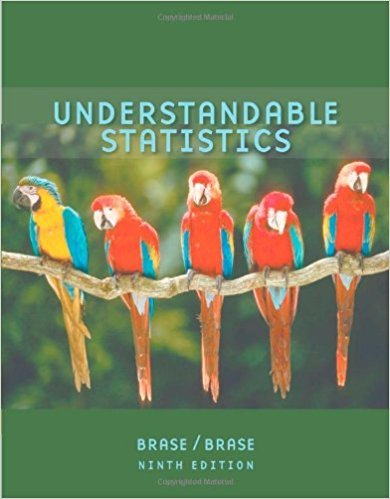×
×

# Civil Engineering: Soil Profiles Sand and clay studiesISBN: 9780618949922 213

## Solution for problem 10 Chapter 12.4

Understandable Statistics | 9th Edition

• Textbook Solutions
• 2901 Step-by-step solutions solved by professors and subject experts
• Get 24/7 help from StudySoup virtual teaching assistantsUnderstandable Statistics | 9th Edition

4 5 1 323 Reviews
19
1
Problem 10

Civil Engineering: Soil Profiles Sand and clay studies were conducted at the West Side Field Station of the University of California. (Reference: Professor D. R. Nielsen, University of California, Davis.) Twelve consecutive depths, each about 15 cm deep, were studied and the following percentages of clay in the soil were recorded. 47.4 43.4 48.4 42.6 41.4 40.7 46.4 44.8 36.5 35.7 33.7 42.6 (i) Convert this sequence of numbers to a sequence of symbols A and B, where A indicates a value above the median and B a value below the median. (ii) Test the sequence for randomness about the median. Use a

Step-by-Step Solution:
Step 1 of 3

Stats 401 Week 2 Relative frequency = How many times appeared in category ÷ Total items in category (like finding percent without multiplying by 100 at the end) For the median, if there is an even amount of number given then add the two middle terms then divide by 2. EX: 1 2 3 4 5 6. The two middle terms are 3 and 4 so 3 + 4 = 7. Then 7/2 = 3.5 (finding...

Step 2 of 3

Step 3 of 3

##### ISBN: 9780618949922

Unlock Textbook Solution